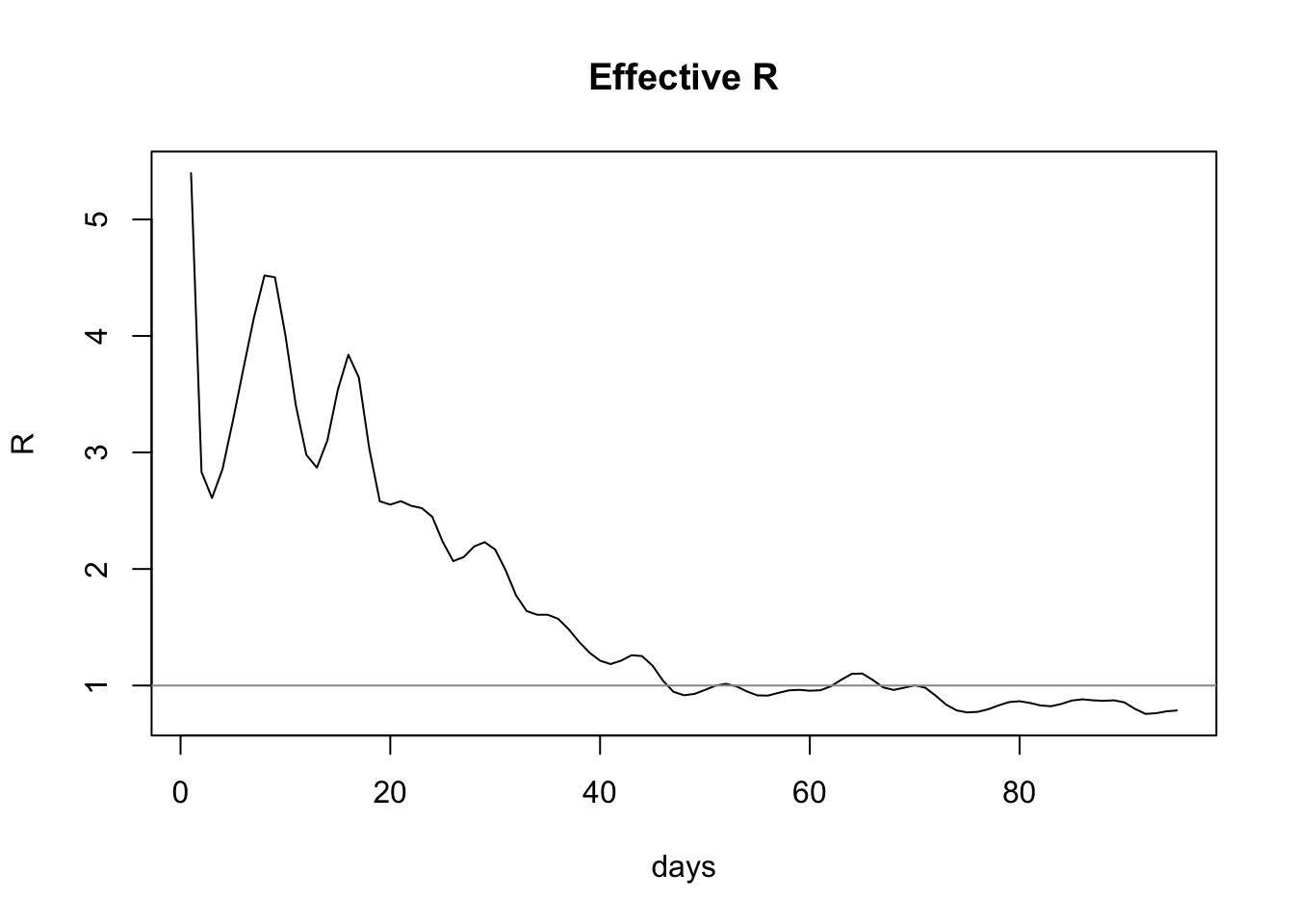I’ve just realised why my estimates of R do not tally with the established figures, especially after the peak. The reason is an absurdly simple case of user error, for which I can only apologise.

The R0 estimate.R function accepts an epidemic curve as its primary data. An epidemic curve is a time series of new cases over time. Unfortunately, I have been using the number of confirmed cases over time. This value only increases, so the value of R it calculates will never fall below 1 (although it may reach 1). This wasn’t that noticeable before the peak, but was definitely a problem after it.

Let’s plot estimated R for the UK again, only this time converting the confirmed case data into a time series of new cases.

``````library(tidyverse)
library(lubridate)
library(R0)``````
``````confirmed <- read.csv(url("https://raw.githubusercontent.com/CSSEGISandData/2019-nCoV/master/csse_covid_19_data/csse_covid_19_time_series/time_series_covid19_confirmed_global.csv"))
confirmed <- subset(confirmed, select = -c(Lat, Long))

ukConfirmed <- confirmed %>%
filter(Country.Region == "United Kingdom")

sums <- colSums(ukConfirmed[,-match(c("Province.State", "Country.Region"), names(ukConfirmed))], na.rm=TRUE)
sumsByDateCode <- as.data.frame(t(t(sums)))
colnames(sumsByDateCode) <- c("count")

dailyCounts <- sumsByDateCode %>%
mutate(day = row_number()) %>%
mutate(new_cases = count - lag(count, default=count)) %>%
filter(count > 0) %>%
filter(new_cases > 0)``````

We’ll continue to use a generation time distribution with a mean of 5 days, standard deviation 1.9:

``meanGenerationTime <- generation.time("gamma", c(5, 1.9))``

Let’s estimate R:

``est <- estimate.R(dailyCounts\$new_cases, end=length(dailyCounts\$new_cases), methods=c("TD"), GT=meanGenerationTime)``
``````estimatedR <- est\$estimates\$TD\$R[1:length(est\$estimates\$TD\$R)-1]

estimateRByDay <- as.data.frame(estimatedR) %>%
rename(R = estimatedR) %>%
mutate(day = row_number())

plot(estimateRByDay\$day, estimateRByDay\$R, xlab="days", ylab="R", type="l", main="Effective R")
abline(h=1, col="gray60")``````And thus we see the most recent value for effective R is 0.7860974, which is in agreement with the published value of R, which at the time of writing is given as 0.7-0.9.

I have since corrected the COVID-19 Explorer to use the correct calculation.